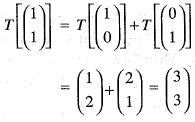Courses

# Test: Linear Algebra - 3

## 20 Questions MCQ Test Topic-wise Tests & Solved Examples for IIT JAM Mathematics | Test: Linear Algebra - 3

Description
This mock test of Test: Linear Algebra - 3 for Mathematics helps you for every Mathematics entrance exam. This contains 20 Multiple Choice Questions for Mathematics Test: Linear Algebra - 3 (mcq) to study with solutions a complete question bank. The solved questions answers in this Test: Linear Algebra - 3 quiz give you a good mix of easy questions and tough questions. Mathematics students definitely take this Test: Linear Algebra - 3 exercise for a better result in the exam. You can find other Test: Linear Algebra - 3 extra questions, long questions & short questions for Mathematics on EduRev as well by searching above.
QUESTION: 1

### The system of equations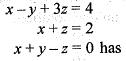Solution:

We are given that the system of equation,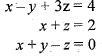It may be written in the matrix form as,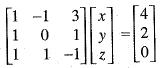Here, the coefficient of matrix is,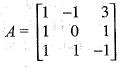and the augmented matrix is given by,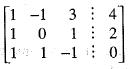Reduce the system equation in echelon form using the operations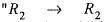R1" and
R3 -> R3 - R1
These operation yield: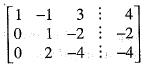and also, R3 --> R3 - 2R2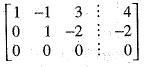Here, Rank of A = Rank of aug(A) < number of unknowns.
Hence, the given system of equation has infinite many solutions.

QUESTION: 2

### ​Which one of the following is true?

Solution:

We need to find the dim Horn  (P3t, R2)
Since dim (P3t) = 3
and dim R2 = 2
Therefore,dim Hom (P3t), R2)
= 3 x 2 = 6

QUESTION: 3

### Which one of the following is true?

Solution:

We need to find the dimension of Horn (M2,3, R4).
Since dim M2,3 = 2 x 3 = 6
and dim R4 = 4
Therefore,dim Horn (M2,3, R4)
= 6 x 4 = 24
Let Mn,m be the vector space of all n x m matrices over R. Then
dim Mn,m = n x m

QUESTION: 4

Let :R3 --> R2 be the linear transformation given by
T(x, y, z) = (x, y),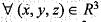with respect to standard basis of R3 and the basis {(1,0), (1, 1)} of R3. What is the matrix representation of T?

Solution:

Let T : R3--> R2 be the linear transformation defined by
T(x, y, z) = (x, y)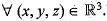we need to determine the matrix of linear transformation T w.r.t. standard basis of R3 and the basis {(1, 0), (1 ,1 } of R2. since {(1 ,0 ,0) , (0,1,0) (0,0,1)} be the standard basis of R3, therefore,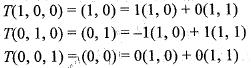Thus, the matrix of linear transformation T w.r.t. the standard basis R3 and the basis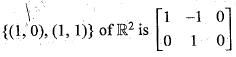QUESTION: 5

Let T : R3 --> R3 be defined by T(x, y, z) = (x, y, 0) and S : R2 —> R2 be defined by S(x, y) = (2x, 3y), be linear transformations on the real vector spaces R3 and R2, respectively. Then, which one of the following statement is correct?

Solution:

Let T : R3 -> R3 be the linear transformation defined by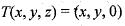and S : R2 ---> R2 be the linear transformation defined by
S(x, y) = (2x, 3y)
Now,ker T = {(x, y , z) such that T(x,y, z) = 0}
= {(x, y ,z) : (x, y, 0) = (0,0,0)}
= {(0,0,z) : z ∈ R }
therefore, ker T ≠ {0, 0, 0} hence T is singular.
Next,ker S = {(x, y ) : S(x, y) = 0}
= {(x,y) : (2x,3y) = (0, 0)}
= {(0, 0)}
Therefore, S is non-singular.

QUESTION: 6

Consider the equation AX = B, where A =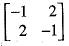and B =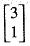, then

Solution:

We are given that the equation AX = B, where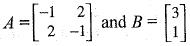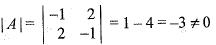Hence, the rank of A = 2 and, the augmented matrix is given by,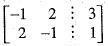The rank of A = rank of aug (A) = 2
= number of unknowns.
Hence, there exist a unique solutions.

QUESTION: 7

The system of equation 2x + y = 5, x - 3y = -1
3x + 4y = k is consistent, when k is

Solution:

We are given that the system of equations,
2x + y = 5
x - 3y = - 1
3x + 4y = k is consistent.
We need to find the value of k. The given system of equation may be written as,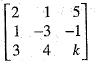Since, this system of equation is consistent. Therefore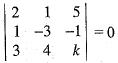or 2(-3k + 4) -1(k +3) + 5(4 + 9) = 0
-7k + 70 = 0
k =10

QUESTION: 8

The value of α for which the system of equations
x + y + z = 0
y + 2z = 0
αx + z = 0 has more than one solution is

Solution:

We are given that the system of equation,
x + y + z =0
y + 2z = 0
αx + z= 0
has more than one solution. We need to find the value of α. The determinant of the coefficient matrix must be zero, i.e.,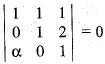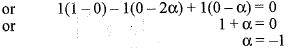QUESTION: 9

The system of the equations:
x + 2y + z - 9
2x + y + 3z=7
can be expressed as

Solution:

We are given that the system of equations,
x + 2y + z = 9
2x + y + 3z = 7
This system of equation can be expressed in the form AX = B i.e.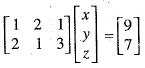which is required matrix form.

QUESTION: 10

Let A be an n x n matrix from the set of numbers and A3 - 3A2 + 4A - 6I = 0, w hereI is nxn unit matrix. If A-1 exist, then

Solution:

We are given that A be an n x n matrix from the set of real num bers and A3 - 3A2 + 4A - 6I = 0 ,
where, I is n x n unit matrix.
Since,
A3 - 3A2 + 4A = 6I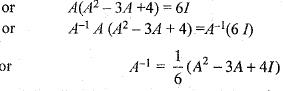QUESTION: 11

If T : R2 --> R3 is a linear transformation T(1, 0) = (2, 3, 1) and T(1,1) = (3,0,2), then which one of the following is correct?

Solution:

We are given that a linear transformation T: R2--> R3
show that
T(1,0) = (2,3,1)
and T(1 , 1) = (3, 0, 2)
We need to determine the image of (x, y) under the linear transformation T.
Let there exist scalars α, β,
such that (x,y) = a(l,0) + β(l, 1) or equivalently
(x,y) =(α + β, β)
Comparing the components of the co-ordinates we get,
α + β = x, β = y
Solving for α and β, we get
α = x-y, β = y
therefore, (x, y) = (x - y) (1,0) + y( 1,1) taking the image under linear transformation T, we get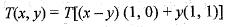Using the linearity condition, we get T(x,y) = (x-y)T(l,0)+yT(l,l)
Substituting the value of T(1,0) and T(1, 1) we get
T(x, y) = (x -y) (2, 3, 1) + y(3, 0, 2)
= (2x -2y + 3y, 3x - 3y, x - y + 2y)
= (2x + y, 3x - 3y, x + y)
Therefore , the image of (x, y) under T that is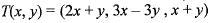QUESTION: 12

Consider, the linear transformation
T : R4 ----> R4 given by:
T(x, y, z, u) = (x, y, 0, 0),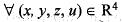Then, which one of the following is correct?

Solution:

We are given that linear transformation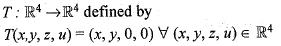We need to determine Rank and Nullity of T.
Let (x,y, z, u) ∈ ker T
Then T(x, y, z, u) = (0, 0, 0, 0)
Using the definition of linear transformation we get
(x,y, 0, 0) = (0, 0, 0, 0)
implies x = 0, y = 0, z and u are arbitrary
Therefore,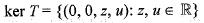Hence, Nullity of T = 2
Using Rank Nullity theorem, we get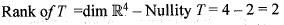QUESTION: 13

What is the rank of the linear transformation T : R3 ---> R3 defined by T(x, y, z) = (y, 0, z)?

Solution:

We are given that the linear transformation T : R3 ---> R3 defined by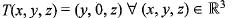We need to determine the rank of the linear transformation T.
Let (x, y, z) ∈ ker T
ThenT(x, y, z) = (0, 0, 0)
Using the definition of linear transformation, we get,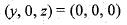implies y = 0, z = 0 and x is arbitrary Therefore, ker T = {( x, y, z ) : y = 0, z = 0 and x is arbitrary}
Hence, Nullify of T= 1
Using Rank Nullity theorem, we get Rank T= dim R3 - Nullity T
= 3 - 1 = 2

QUESTION: 14

Let V be the vector space of all 2 x 2 matrix over the field R of real numbers and B =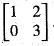. If T : V--> V is a linear transformation defined by T(A) = AB - BA, then what is the dimension of the Kernel of T?

Solution:

Let V be the vector space of all 2 x 2 matrices over the field R of real numbers and matrix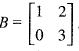Let T : V —> V be a linear transformation defined by T(A) = AB - BA
We need to determine the dim of ker T.
Let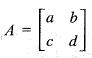Then, take T(A) = 0 implies AB - BA = 0
Substituting the values of matrices A and B,
we get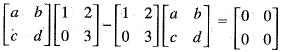or equivalently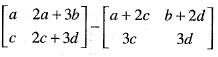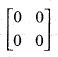or equivalently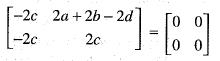implies c =0 and a + b - d = 0 Therefore,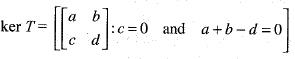Hence, dim (ker T) = Total number of variables - Number of restrictions = 4 - 2 = 2

QUESTION: 15

Let T :R3 ---> R3 be a linear transformation given by T(x, y, z)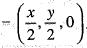. What is the rank of T?

Solution:

We are given that the linear transformation T : R3---> Rdefined by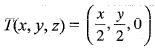We need to determine Rank of T.
Now,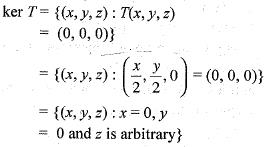Therefore, dim of ker T = 1
Hence by Rank Nullity theorem, we get Rank T = dim R3 - Nullity T
= 3 - 1 = 2

QUESTION: 16

Let T : R3 ---> R3 be a linear transformation given by T(x, y, z) = (x, y, 0). Then, the null space is generated by which one of the following?

Solution:

We are given that a linear transformation T : R3 —> R3 defined by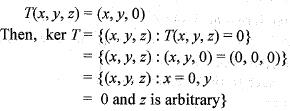Therefore
(0,0,1) ∈ ker T and Hence, the Null space is generated by (0, 0,1).

QUESTION: 17

Consider the vector space C over R and let 7: C --> C be a linear transformation given by T(z) = z. Then, which one of the following is correct?

Solution:

We are given that a linear transformation T : C -->C defined by T(z) =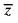Where C is a vector space over the field of Real Number R and z is the conjugate of complex number z.
ker T= {z : T(z) = 0}
= { z : z = 0} = {0}
Hence, T is one-one.
Since C over R is a finite dimensional vector space (two dimensional vector space) and T : C --> C is one linear transformation. Therefore T is onto.

QUESTION: 18

The rank of the matrix (m × n) where m<n cannot be more than?

Solution:

let us consider a 2 × 3 matrix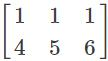Where R1 ≠ R2 rank is 2
Another 2 × 3 matrix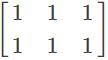Here, R1 = R2 rank is 1
And the rank of these two matrices is 1, 2
So rank cannot be more than m.

QUESTION: 19

Consider the mapping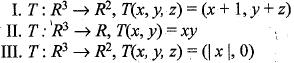Q. ​Which of the above are linear transformation?

Solution:

I. We are given that the transformation T: R3 —> R2 defined by
T (x , y , z) = (x + 1, y + z)
We need to determine the linearity of the given linear transformation.
Now,T'(0, 0, 0) = (1, 0)
Since the image of (0, 0,0) under transformation T is (1, 0) which is not the zero of R2.
Hence, T is non-linear.
II. We are given that the transformation T : R3 --> R defined by
T(x,y,z) = (xy)
We need to determine the linearity of this given transformation.
Since the image of (x, y, z) under linear transformation.
T is an algebraic term of degree 2.
Therefore, T is non linear.
III. We are given that the transformation T: R3 —> R2 defined by
T(x, y, z) = (| x |, 0)
We need to determine the linearity of this linear transformation
Let (1, 0, 0) and (-1, 0, 0) be two vectors of R3
Then T(l,0,0) = (l,0)
andT(-l, 0, 0) = (1, 0)
Now,T [(1, 0, 0) + (-1, 0, 0)] =
T(0, 0, 0) = (0, 0)
and T(1, 0, 0) + T(-1, 0, 0)
= (1, 0) + (1,0) = (2, 0)
Hence, T [(1, 0, 0) + (-1, 0, 0)] ≠ T{ 1, 0, 0) + T (-1, 0, 0).
Therefore, T is non linear.

QUESTION: 20

Let T : R2 --->R2 be a linear transformation such that T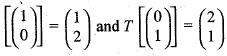What is the value of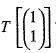?

Solution:

Let T : R2 —> R2 be a linear transformation such that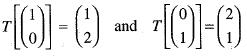We need to determine the image of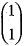under linear tansformation T.
Let there exist scalars α and β such that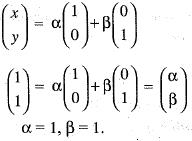Since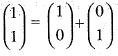and taking the image under T, we get